AtomicDensityMatrix`
AtomicDensityMatrix`

# HyperfineA

HyperfineA

is a parameter for AtomicState that parameterizes the hyperfine splitting of the state due to the electronic interaction with the nuclear magnetic dipole moment.

HyperfineA[state]

returns the value specified for HyperfineA in AtomicState state, or HyperfineA[StateLabel[state]] if it is not specified.

# Details and Options

• HyperfineA is considered to have units of angular frequency.
• The hyperfine shift of a state with HyperfineA and HyperfineB denoted by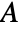and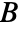, respectively, is given by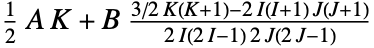, where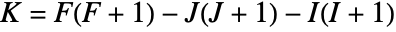and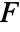,, andare the total, total electronic, and total nuclear angular-momentum quantum numbers, respectively.
• HyperfineA is automatically threaded over a list of AtomicState objects appearing as its argument.

# Examples

## Basic Examples(1)

Specify the HyperfineA parameter for a state with hyperfine structure:

Get the specified HyperfineA parameters for each sublevel:

The value of HyperfineA is used to calculate the hyperfine shift for each sublevel: